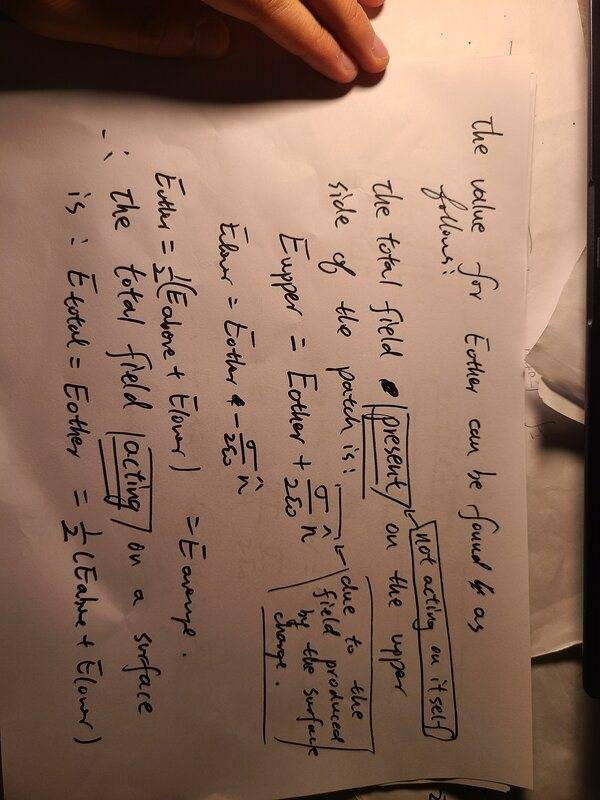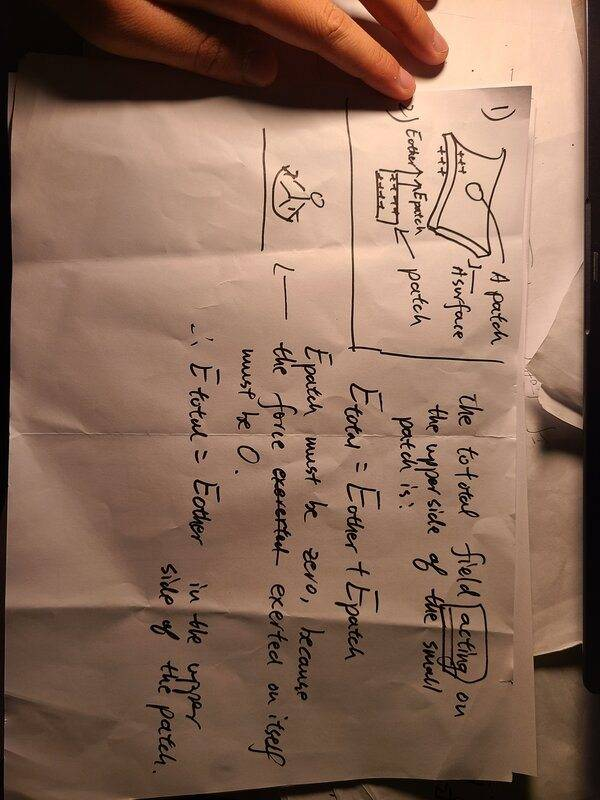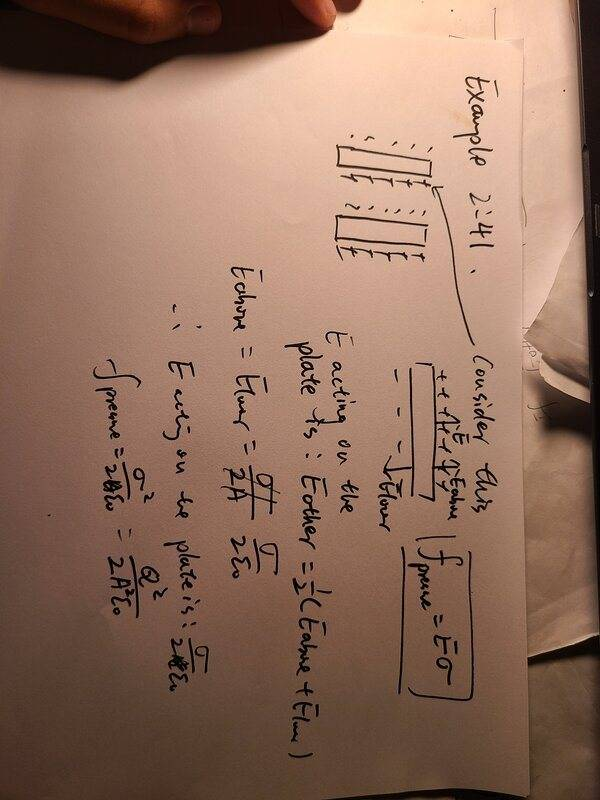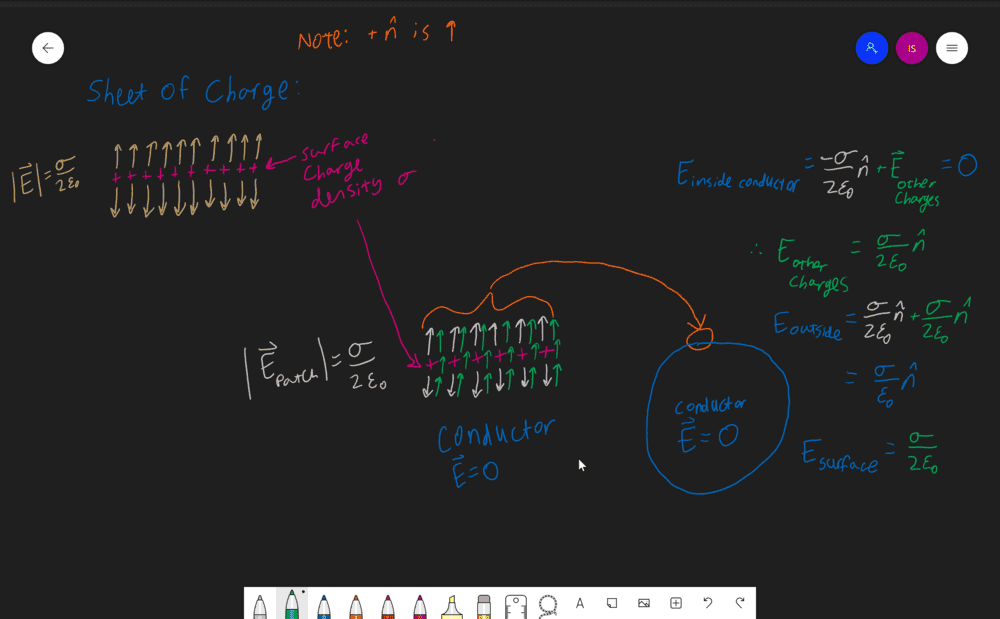# What is the meaning of electric field "immediately outside the surface"?

Tony Hau
Today when I am reading Griffith's electrodymamics on surface charge and force on conductors, I have come across two very ambiguous terms: electric field at the surface and immediately outside the surface.

The context of these two words is as follows:
The electric field immediately outside is equal to sigma/epsilon(normal vector pointing outwards).

Then, the force per unit area is equal to sigma times the electric field at the surface.

I am pretty confused here about the difference between electric field at the surface and immediately outside.

The explanation comes from this video, not the original book, although the video is only trying to explain the chapter on Griffith's book.

As Griffiths states, the electric field directly above or below the surface is caused by two things: the charged patch itself (##E_{patch}=\frac{\sigma}{2\epsilon_0}##) and any other electric field not related to the patch (##E_{other}##). That is ##E_{near surface}=E_{other}+ E_{patch}##, where the sign of ##E_{patch}## depends on whether we are talking about the field above or below the surface.

The idea is that the surface charge cannot exert a force on itself-- thus, the field AT the surface cannot be caused by the patch. Evidently, ##E_{at surface}=E_{other}##. If there is no other electric field (as in the case of an isolated sheet of charge), then there is no electric field at the surface, while the electric field right outside the surface remains ##\frac{\sigma}{2\epsilon_0}##.

In the case of the conductor, there is another force-- remember, all of the charges are repelling each other. Using the fact that the field inside the conductor is zero, you can show that due to the equation ##E_{near surface}=E_{other}+ E_{patch}##, the "other" field must be ##\frac{\sigma}{2\epsilon_0}##, and that's the field at the surface of the conductor, as it is only the field caused by the other charges on the conductor.

I hope this clears up some of your confusion.

Tony Hau
As Griffiths states, the electric field directly above or below the surface is caused by two things: the charged patch itself (##E_{patch}=\frac{\sigma}{2\epsilon_0}##) and any other electric field not related to the patch (##E_{other}##). That is ##E_{near surface}=E_{other}+ E_{patch}##, where the sign of ##E_{patch}## depends on whether we are talking about the field above or below the surface.

The idea is that the surface charge cannot exert a force on itself-- thus, the field AT the surface cannot be caused by the patch. Evidently, ##E_{at surface}=E_{other}##. If there is no other electric field (as in the case of an isolated sheet of charge), then there is no electric field at the surface, while the electric field right outside the surface remains ##\frac{\sigma}{2\epsilon_0}##.

In the case of the conductor, there is another force-- remember, all of the charges are repelling each other. Using the fact that the field inside the conductor is zero, you can show that due to the equation ##E_{near surface}=E_{other}+ E_{patch}##, the "other" field must be ##\frac{\sigma}{2\epsilon_0}##, and that's the field at the surface of the conductor, as it is only the field caused by the other charges on the conductor.

I hope this clears up some of your confusion.

#### Attachments

Tony Hau
I have included two pictures above to illustrate my reasoning.

Actually I don't know what the difference between a conductor and a surface is. In my imagination, a surface is merely a thin conductor, in which the upper surface and lower surface is filled with charges. What makes it special for conductor not to have a zero E patch force on itself? If you are pleased, it is very good to draw a diagram to illustrate the things you are talking about.

Sorry I really feel that I am stupid!

Gold Member
2022 Award
I think this is indeed a bit confusing. I guess what Griffiths really wants to say are the boundary conditions across singularities like the surface of a conductor. This generalizes the differential operators div and rot to such singular cases.

For that you have to remember the covariant definition of div and rot as specific limits of integrals. Let's take the divergence of a vector field at a point ##\vec{x}##. It's calculated by defining small volumes ##V## containing the point ##\vec{x}## and then defining
$$\vec{\nabla} \cdot \vec{E}(\vec{x})=\lim_{V \rightarrow \{\vec{x}\}} \frac{1}{V} \int_{\partial V} \mathrm{d}^3 r \vec{E}(\vec{r}),$$
where the limit means that one shrinks the volume to the point ##\vec{x}##. If this limit doesn't depend on the specific volumes you have a vector field, where the divergence exists, and it is easy to show that for Cartesian components you get
$$\vec{\nabla} \cdot \vec{E}=\partial_i E_i,$$
using the Einstein summation convention. The divergence of a vector field is its source, and that's why Gauß's Law reads
$$\vec{\nabla} \cdot \vec{E}=\rho.$$
This of course does not work anymore for singular charge distributions like a surface charge, because in such a case the limit defining the divergence does not exist to begin with. In this case a related quantity exists, the surface divergence. Here you define
$$\mathrm{Div} \vec{E}(\vec{x}) = \frac{1}{S \rightarrow \{\vec{x} \}} \int_S \mathrm{d}^2 \vec{f} \cdot \vec{E}(\vec{r})$$
for any closed surface. Evaluating this across a surface which carries a surface-charge density you get
$$\mathrm{Div} \vec{E}=\vec{n} \cdot (\vec{E}_1-\vec{E}_2)=\sigma.$$
Where ##\vec{E}_1## and ##\vec{E}_2## are the values of ##\vec{E}## in the limit from either side of the singular surface and ##\vec{n}## is the surface normal pointing from side ##2## into side ##1## of the surface.

An alternative is to use always volume charge density which become singular like a Dirac ##\delta## distribution across the singular surface. Both definitions are equivalent.

The same arguments lead to the definition of a surface curl, using surfaces and their boundary analogously as you use volumes and their boundary to define the divergence and surface divergence.

••Motocross9 and etotheipi
Homework Helper
Gold Member
"I am pretty confused here about the difference between electric field at the surface and immediately outside."
The problem is that Griffith's English is ambiguous here. He is talking here about two different electric fields.
The E field 'at the surface', is an external E field exerting a force on the surface charge.
The 'electric field immediately outside' is the E field caused by the surface charge.

•rude man, etotheipi and Tony Hau
Tony Hau
After thoroughly reading Griffith's book, word by word, carefully, I have reached this understanding which I find satisfactory. I am able to solve the two follow up questions on Griffith's book.#### Attachments

Tony Hau
This is one of the questions I have done. The question is about finding the electrostatic pressure on the metal plates, each of which is charged with Q and has an area A.Mentor
@Tony Hau two things:

First, your pictures show up sideways, not right side up. That makes them very hard to read.

Second, please use the PF LaTeX support to post equations, and post text as ordinary text. Images should only be used for actual drawings--things that cannot be posted in one of those two ways. If you need help with the PF LaTeX features, there is a "LaTeX Guide" link at the lower left of the edit box that is used for posts.

•Tony Hau
Something seems a little off with those pictures, so I figured I would add a picture with my work for this. Note that the only reason I am including equations in the picture is so that they are color-coded, so that you can match the terms to the vector on the diagram:Notice that for the sheet of charge, there is no electric field AT the surface-- unless there is some external electric field that I did not draw, in which case the field at the surface would just be that external field. For an isolated conductor, there must be an electric field caused by the other charges in the conductor (i.e. on the other sides) that makes it so the field inside the conductor is zero (note that my diagram shows what is happening immediately above and below the surface, so the field caused by the other charges is constant). You'll notice that there is no field caused by the patch (the silver vectors) right at the surface-- thus the electric field at the surface is only the (green) field caused by the other charges in the conductor.

For me, the field lines help me understand it better-- I know it may not be the same for everyone.

•Tony Hau
Homework Helper
Gold Member
Actually I don't know what the difference between a conductor and a surface is. In my imagination, a surface is merely a thin conductor, in which the upper surface and lower surface is filled with charges. What makes it special for conductor not to have a zero E patch force on itself? If you are pleased, it is very good to draw a diagram to illustrate the things you are talking about.
A surface has zero depth, by definition. A charge layer is assumed to have zero depth because the charges (electrons and ions) are assumed to be points.

You can have surface charge on the sides of any conductor.

Incidentally, when a problem says 'conductor' in electrostatics it can comprise any finite-resistivity medium. The E field of any finite-resistivity (< ##\infty##) is therefore zero. If the medium has high resistivity it may take some time for the charges to aggregate on the surface but eventually they will.

It's different if there are currents present. Then an E field will be finite unless you really have a perfect conductor (zero resisitivity).

Also, the statement "the surface charge cannot exert a force on itself " is perhaps misleading. Every charge on a charged surface exerts a force on every other charge on that surface. That's how all the charges assemble on the surface - they'rfe all trying to avoid each other.

Also, the statement "the surface charge cannot exert a force on itself " is perhaps misleading. Every charge on a charged surface exerts a force on every other charge on that surface. That's how all the charges assemble on the surface - they'rfe all trying to avoid each other.
Although the specific patch does not exert a force on itself. It’s the other charges on the other sides of the conductor that create the pressure.

Homework Helper
Gold Member
Although the specific patch does not exert a force on itself. It’s the other charges on the other sides of the conductor that create the pressure.
Every charge exerts a force on every other charge in any "patch" (surface charge layer). Right thru the conductor itself, from one side to the opposite and everywhere inbetween!

There are actually practically an infinite number of electric fields within a conductor, set up by practically an infinite number of discrete charges, all acting against each other to effect a zero net field.

Mentor
There are actually practically an infinite number of electric fields within a conductor, set up by practically an infinite number of discrete charges, all acting against each other to effect a zero net field.

I have found it helpful to think of a conductor (an idealized one) this way: since charges can move freely inside a conductor, any nonzero net electric field would instantly cause charges to move so as to cancel it out. The only possible equilibrium state is zero net electric field everywhere in the conductor.

Homework Helper
Gold Member
I have found it helpful to think of a conductor (an idealized one) this way: since charges can move freely inside a conductor, any nonzero net electric field would instantly cause charges to move so as to cancel it out. The only possible equilibrium state is zero net electric field everywhere in the conductor.
Exactly.

One thing some folks have difficulty with is the idea of simultaneous existence of electric fields within a conductor that in toto cancel each other to effect the zero net E field.